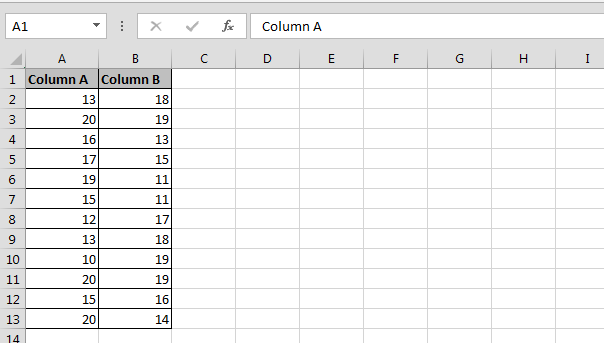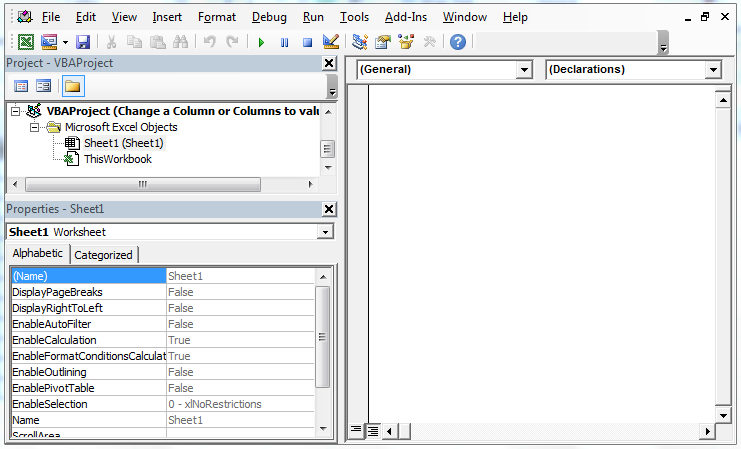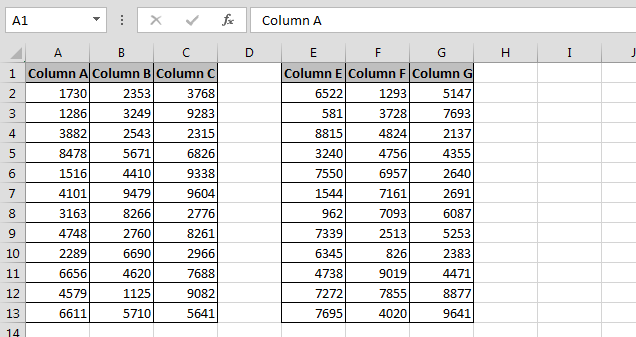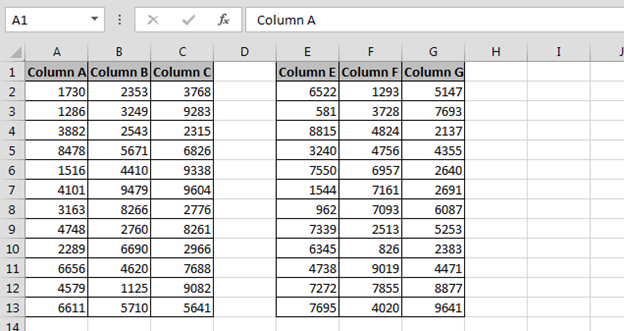# Change a Column or Columns to values using VBA in Microsoft Excel

In this chapter you will learn, how to change all the cells in the active sheet to values, using VBA in Microsoft Excel.

Let’s take an example and understand how we write the VBA code.

How to change the value from column to column?

We have data in Excel in which Column A and column B contains some values, now we want to replace values in Column A values with the values in column B.• Press Alt+F11 key to open the Visual Basic Application• In VBAProject Double click on Sheet 1
• Enter the below given VBA Code
```Sub Values_8()

Columns("A").Value = Columns("B").Value

End Sub
```

• To run the code press F5 key
• The value of column B will get update in Column AHow to change the value from columns to columns?

We have data in Excel in which Column A, B and C contains some values in a group and Column E, F and G contain values in 2nd group, now we want to change the value of 1st group with the 2nd group.To change the value follow below given steps and code:

• Press the key Alt+F11 and double click on sheet 2
• Enter the below code:
```Sub Values_9()

Columns("A:C").Value = Columns("E:G").Value

End Sub
```

• Run the code by pressing the F5
• Values will get updated